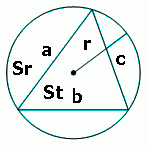# Circumcircle of a triangle

This online calculator determines the radius and area of the circumcircle of a triangle given the three sidesYet another triangle calculator for those who needed radius of triangle circumcircle.
Radius can be found like this:

where S, area of triangle, can be found using Hero's formula

The calculator determines radius and having a radius, area of the circumcircle, area of the triangle, and area ratio - just for reference#### Circumcircle of a triangle

Digits after the decimal point: 2